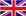English
Blog

### Briefly description of zero phase current transformer

July 10 , 2020

The general current transformer can convert the primary current with a large value to a secondary current with a smaller value according to a certain transformation ratio, so that it can be used for maintenance and accurate measurement. The main function of the zero-sequence current transformer is to protect the operation and disconnect the switching power supply when an electric shock accident or a common leakage fault occurs in the power circuit. Although the names of these two transformers are very similar, their role is very large. Not the same, what is the difference and their main function? The through-type current transformer as a zero-sequence transformer has no problem in terms of working principle, but the sensitivity of the transformer will be very different.

1. The main function of general current transformer

In general, current transformers can convert primary currents with large values to secondary currents with smaller standard values according to a certain transformation ratio, thereby achieving main purposes such as maintenance and accurate measurement. For example, a current transformer with a transformation ratio of 400/5 can change the current of 400A to 5A.

2. The working principle of the zero sequence transformer

The working principle of zero-sequence current maintenance is based on Kirchhoff's current law: the algebraic sum of the complex current injected into any connection point in the power circuit is equal to zero. Under the normal conditions of the line and electrical equipment, the vector sum of the currents of each phase is equal to zero. Therefore, the secondary winding of the zero-sequence current transformer does not have a signal output, and the implementing components will not move. When the common fault of the grounding device occurs, the vector sum of each phase current is not zero. The common fault current will cause magnetic flux in the ring core of the zero sequence current transformer. The induced voltage on the secondary side of the zero sequence current transformer will cause the execution The components carry out actions, drive the tripping equipment, and transform the power supply network, so as to achieve the purpose of ground fault protection.

3. What is the role of zero sequence transformer

When there is an electric shock accident or a common fault of leakage current in the power circuit, it can play a protective action and disconnect the switching power supply.

4. What are the operating conditions of the zero sequence transformer

You can install a current transformer on the three-phase line, or let the three-phase transmission lines cross the zero-sequence current transformer together. Of course, you can also install a zero-sequence current transformer on the neutral point N. The zero-sequence current transformer can be used to check the three-phase current vector sum.

For the practical application of zero-sequence current protection, a current transformer (CT) can be installed on each of the three-phase lines, or the three-phase transmission lines can cross the zero-sequence CT together, and a zero-sequence CT can also be installed on the neutral point N. We can use this zero-sequence CT to check the three-phase current vector sum, that is, the zero-sequence current Io, IA+IB+IC=IO, when the three-phase loads connected to the line are completely equal (common in the absence of grounding devices Failure, and does not consider the leakage current of the line and electrical equipment), IO=0; when the three-phase load connected to the line is unbalanced, then IO=IN, then the zero-sequence current is the unbalanced current IN; when a certain When a common fault of the grounding device occurs on one phase, a single-phase grounding common fault current Id will be generated. At this time, the zero-sequence current IO=IN+Id detected by your friends is actually a three-phase unbalanced current and a single-phase grounding current Vector sum.

#### Tags

welcome to ZTC
Talk To ZTC Today To Discuss Your Need For Current Transformers,Get Standard Item, OEM/ODM Service Quote Are Available.